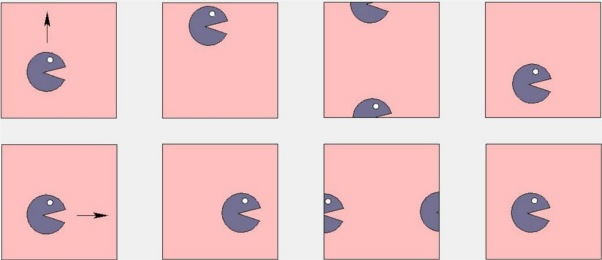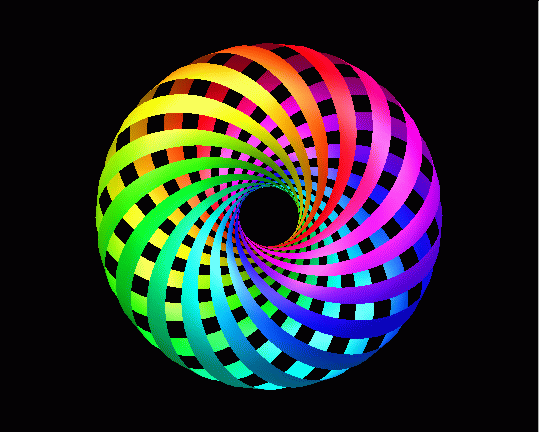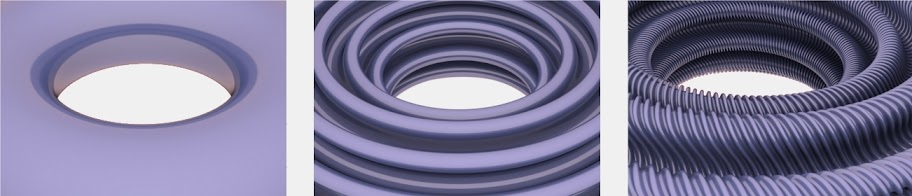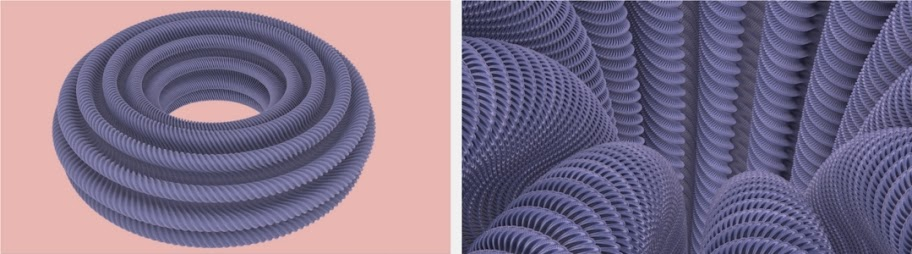### A flat torus in three dimensional space

Vincent Borrelli, Said Jabrane, Francis Lazarus and Boris Thibert realize for the first time the image of a 3d flat torus. But, what is a flat torus? In a mathematical point of view is a torus without gaussian curvature everywhere. For example: in a 3d space, you can bend a sheet of paper in a cylinder, but you cannot embed it in a torus without stretching the paper itself. But, if you live in a 2d space, a flat torus is a surface like the pacman labirint: you can enter in the right side of the paper and exit behind you from the left side:We can define a flat torus starting from a couple of real numbers $u$, $v$ such that $u, v \in ]0, 2 \pi]$(1): $(u, v) \rightarrow \frac{1}{\sqrt{2}} \left ( \cos (u+v), \sin (u+v), \cos (u-v), \sin (u-v) \right )$ The following is the best visualization of a flat torus before Borelli-Jabrane-Lazarus-Thibert:The mathematical problem around the flat torus was challenged by Nash and Kuiper in 1950s:
Nash and Kuiper proved the existence of a representation that does not perturb the lenghts in the square flat torus. For a long time, this existence remained a challenge for the imagination of mathematicians. But proving and showing should sometimes be clearly distinguished in Mathematics. This is well explained by the thief allegory: Let us assume that a group of people is gathered around a jewel in a closed room. Let us further suppose that the light is turned off for a moment and that the jewel has disappeared when the light is again turned on. We then have the proof that a robber is hiding among the attendance but he can not be exhibited. Although the proofs of Nash and Kuiper are much more than an «existential» trap, their proofs do not provide a sufficiently explicit procedure that would allow for visualization or simply for a mental picture of a square flat torus.(2)
Between 70s and 80s the Abel Prize Gromov extracted a method from the work of Nash and Kuiper, proposing the so called convex integration, a very useful tool. Indeed it
(...) does not only yield the existence of a solution, it also provides us with an effective construction.(2)
Starting from this method, Borelli, Jabrane, Lazarus and Thibert realize an algorithm in order to picture the flat torus.
Mathematicians were puzzled by the works of Nash and Kuiper. These works could indeed prove the existence of objects whose regularity was problematic, if not paradoxical. They had to be smooth and rough at the same time... In effect, the mathematical analysis of the images reveals a surface belonging to two antagonist worlds; the smooth surfaces and the fractals,infinitely broken. When zooming in, we invariably observe ripples at smaller and smaller scales. Each ripple - called a corrugation – appears smooth when viewed alone, but the accumulation of those creates an object with a rough and fractal aspect.(2)And in conclusion
Demonstrating that convex integration can be implemented open new perspectives in applied mathematics, notably for solving differential systems originating from Physics and Biology.
More specifically, our images reveal a class of objects whose structure lies inbetween smooth surfaces and fractals. Such objects could play a central rôle for shape analysis. They could also resolve some unexplained paradoxes.(2)

(1) The Flat Torus in the Three-Sphere
(2) A flat torus in three dimensional space (pdf) | Hevea Project

Borrelli, V., Jabrane, S., Lazarus, F., & Thibert, B. (2012). Flat tori in three-dimensional space and convex integration Proceedings of the National Academy of Sciences DOI: 10.1073/pnas.1118478109

#### 1 comment:

1.A slight correction: The colourful illustration of the torus is just the standard way to depict a torus, but is nowhere near to being a flat torus. On the contrary: In this picture we can clearly see the positively curved regions (as on the top of the picture, where the surface curves down in all directions and looks like part of a sphere) and the negatively curved regions (imagine standing "inside" the hole, where the surface is curved like a Pringles potato chip).

Markup Key:
- <b>bold</b> = bold
- <i>italic</i> = italic
- <a href="http://www.fieldofscience.com/">FoS</a> = FoS